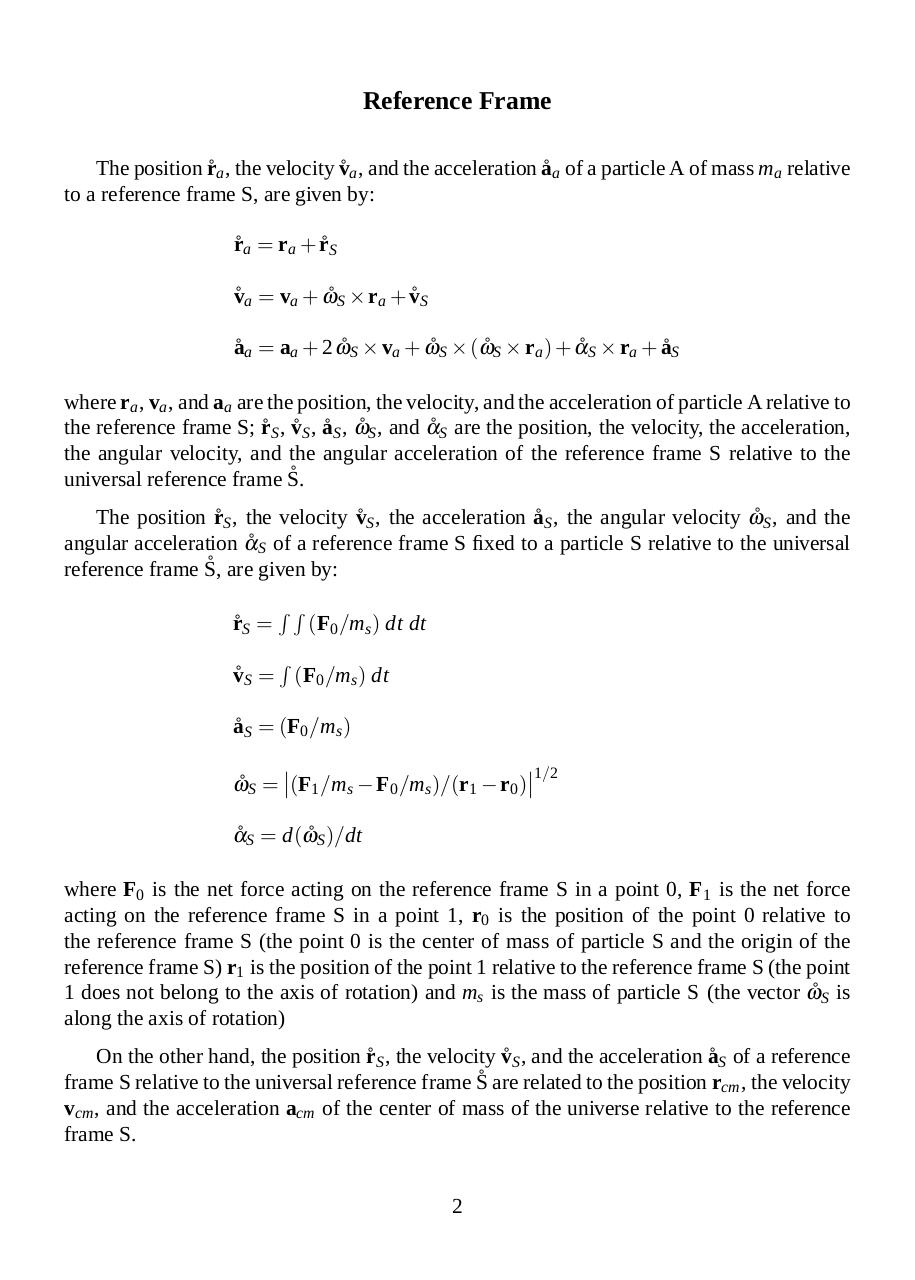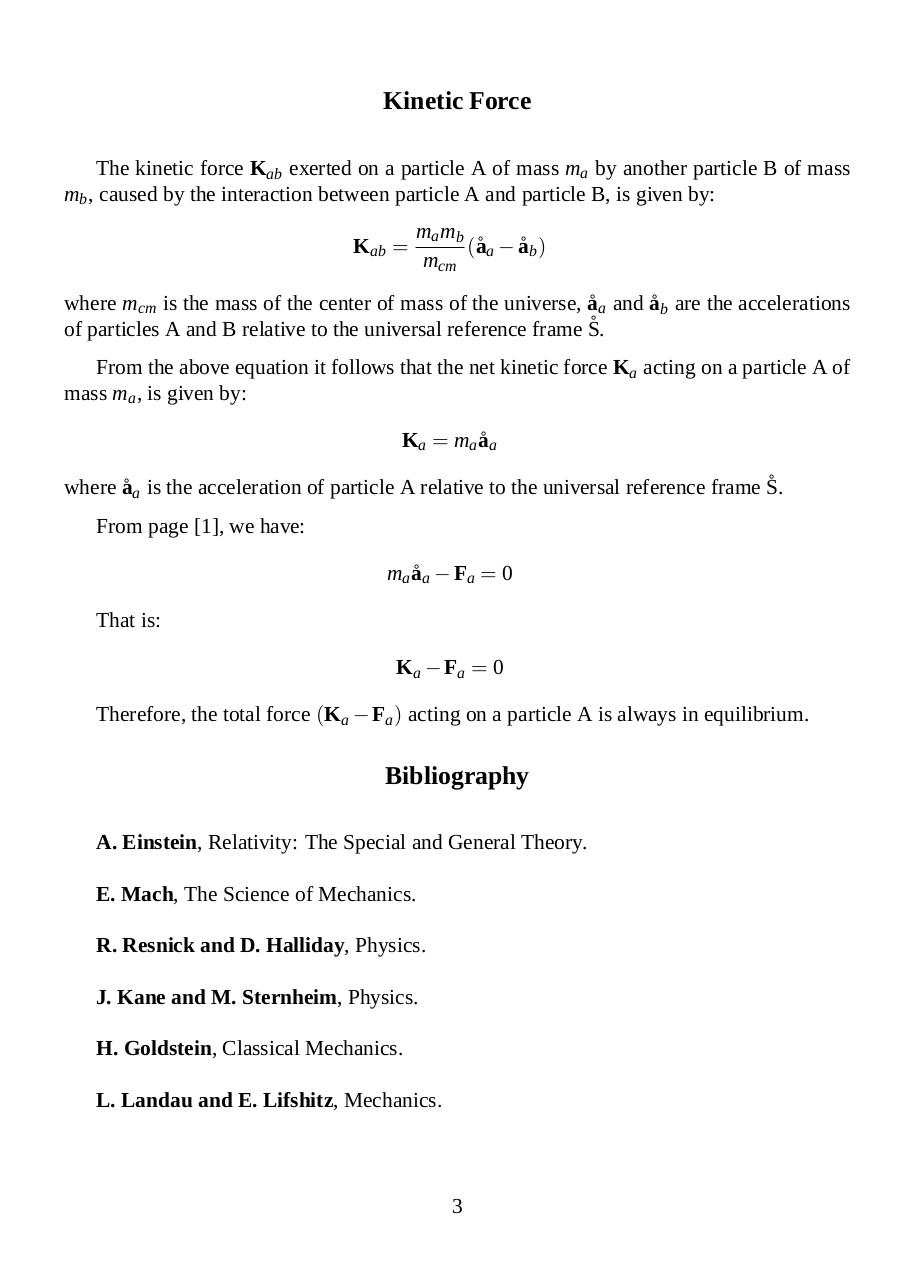## report4 .pdf

Nombre del archivo original: report4.pdf
Título: Universal Reference Frame
Autor: Alejandro A. Torassa

Este documento en formato PDF 1.4 fue generado por LaTeX with hyperref package / pdfeTeX-1.20a, y fue enviado en caja-pdf.es el 11/03/2013 a las 16:23, desde la dirección IP 190.225.x.x. La página de descarga de documentos ha sido vista 1669 veces.
Tamaño del archivo: 25 KB (3 páginas).

### Vista previa del documento

Universal Reference Frame
Alejandro A. Torassa
(2013) Buenos Aires, Argentina
atorassa@gmail.com
Abstract
In classical mechanics, this paper presents the universal reference frame.

Universal Reference Frame
The universal reference frame is a reference frame fixed to the center of mass of the
universe.
The position r˚ a , the velocity v˚ a , and the acceleration a˚ a of a particle A of mass ma relative
˚ are given by:
to the universal reference frame S,
r˚ a =

RR

(Fa /ma ) dt dt

R

v˚ a = (Fa /ma ) dt
a˚ a = (Fa /ma )
where Fa is the net force acting on particle A.
From the above equations the following equations are obtained:
ma r˚ a −

RR

Fa dt dt = 0

2
1/2 m r
a ˚a

− 1/2 ma (

%

ma a˚ a − Fa = 0

where 1/2 v˚ a2 =

R

(Fa /ma ) dt dt)2 = 0

ma v˚ a − Fa dt = 0

R

RR

a˚ a d r˚ a → 1/2 ma v˚ a2 =

2
1/2 m v
a ˚a

R

− Fa d r˚ a = 0

2
1/2 m a
a ˚a

R

− 1/2 ma (Fa /ma )2 = 0

ma a˚ a d r˚ a → 1/2 ma v˚ a2 =
1

R

Fa d r˚ a

Reference Frame
The position r˚ a , the velocity v˚ a , and the acceleration a˚ a of a particle A of mass ma relative
to a reference frame S, are given by:
r˚ a = ra + r˚ S
v˚ a = va + ω˚ S × ra + v˚ S
a˚ a = aa + 2 ω˚ S × va + ω˚ S × (ω˚ S × ra ) + α˚ S × ra + a˚ S
where ra , va , and aa are the position, the velocity, and the acceleration of particle A relative to
the reference frame S; r˚ S , v˚ S , a˚ S , ω˚ S , and α˚ S are the position, the velocity, the acceleration,
the angular velocity, and the angular acceleration of the reference frame S relative to the
˚
universal reference frame S.
The position r˚ S , the velocity v˚ S , the acceleration a˚ S , the angular velocity ω˚ S , and the
angular acceleration α˚ S of a reference frame S fixed to a particle S relative to the universal
˚ are given by:
reference frame S,
r˚ S =

RR

(F0 /ms ) dt dt

R

v˚ S = (F0 /ms ) dt
a˚ S = (F0 /ms )

1/2
ω˚ S = (F1 /ms − F0 /ms )/(r1 − r0 )
α˚ S = d(ω˚ S )/dt
where F0 is the net force acting on the reference frame S in a point 0, F1 is the net force
acting on the reference frame S in a point 1, r0 is the position of the point 0 relative to
the reference frame S (the point 0 is the center of mass of particle S and the origin of the
reference frame S) r1 is the position of the point 1 relative to the reference frame S (the point
1 does not belong to the axis of rotation) and ms is the mass of particle S (the vector ω˚ S is
along the axis of rotation)
On the other hand, the position r˚ S , the velocity v˚ S , and the acceleration a˚ S of a reference
frame S relative to the universal reference frame S˚ are related to the position rcm , the velocity
vcm , and the acceleration acm of the center of mass of the universe relative to the reference
frame S.
2

Kinetic Force
The kinetic force Kab exerted on a particle A of mass ma by another particle B of mass
mb , caused by the interaction between particle A and particle B, is given by:
Kab =

ma mb
(˚aa − a˚ b )
mcm

where mcm is the mass of the center of mass of the universe, a˚ a and a˚ b are the accelerations
˚
of particles A and B relative to the universal reference frame S.
From the above equation it follows that the net kinetic force Ka acting on a particle A of
mass ma , is given by:
Ka = ma a˚ a
˚
where a˚ a is the acceleration of particle A relative to the universal reference frame S.
From page , we have:
ma a˚ a − Fa = 0
That is:
Ka − Fa = 0
Therefore, the total force (Ka − Fa ) acting on a particle A is always in equilibrium.

Bibliography
A. Einstein, Relativity: The Special and General Theory.
E. Mach, The Science of Mechanics.
R. Resnick and D. Halliday, Physics.
J. Kane and M. Sternheim, Physics.
H. Goldstein, Classical Mechanics.
L. Landau and E. Lifshitz, Mechanics.

3